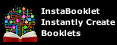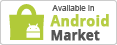Subject:

# Kinematics

Classical mechanics History Timeline Applied Celestial Continuum Dynamics Kinematics Kinetics Statics Statistical Acceleration Angular momentum Couple D'Alembert's principle Energy kinetic potential Force Frame of reference Impulse Inertia / Moment of inertia Mass Mechanical power Mechanical work Moment Momentum Space Speed Time Torque Velocity Virtual work Analytical mechanics Lagrangian mechanics Hamiltonian mechanics Routhian mechanics Hamilton–Jacobi equation Appell's equation of motion Udwadia–Kalaba equation Damping (ratio) Displacement Equations of motion Euler's laws of motion Fictitious force Friction Harmonic oscillator Inertial / Non-inertial reference frame Mechanics of planar particle motion Motion (linear) Newton's law of universal gravitation Newton's laws of motion Relative velocity Rigid body dynamics Euler's equations Simple harmonic motion Vibration Circular motion Rotating reference frame Centripetal force Centrifugal force reactive Coriolis force Pendulum Tangential speed Rotational speed Angular acceleration / displacement / frequency / velocity Galileo Newton Kepler Horrocks Halley Euler d'Alembert Clairaut Lagrange Laplace Hamilton Poisson Daniel Bernoulli Johann Bernoulli Cauchy v t e Kinematics is the branch of classical mechanics which describes the motion of points (alternatively "particles"), bodies (objects), and systems of bodies without consideration of the masses of those objects nor the forces that may have caused the motion. Kinematics as a field of study is often referred to as the "geometry of motion" and as such may be seen as a branch of mathematics. Kinematics begins with a description of the geometry of the system and the initial conditions of known values of the position, velocity and or acceleration of various points that are a part of the system, then from geometrical arguments it can determine the position, the velocity and the acceleration of any part of the system. The study of the influence of forces acting on masses falls within the purview of kinetics. For further details, see analytical dynamics.Kinematics is used in astrophysics to describe the motion of celestial bodies and collections of such bodies. In mechanical engineering, robotics, and biomechanics kinematics is used to describe the motion of systems composed of joined parts (multi-link systems) such as an engine, a robotic arm or the skeleton of the human body.The use of geometric transformations, also called rigid transformations, to describe the movement of components of a mechanical system simplifies the derivation of its equations of motion, and is central to dynamic analysis.Kinematic analysis is the process of measuring the kinematic quantities used to describe motion. In engineering, for instance, kinematic analysis may be used to find the range of movement for a given mechanism, and working in reverse, using kinematic synthesis used to design a mechanism for a desired range of motion. In addition, kinematics applies algebraic geometry to the study of the mechanical advantage of a mechanical system or mechanism. ^ Edmund Taylor Whittaker (1904). A Treatise on the Analytical Dynamics of Particles and Rigid Bodies. Cambridge University Press. Chapter 1. ISBN 0-521-35883-3.  ^ Joseph Stiles Beggs (1983). Kinematics. Taylor & Francis. p. 1. ISBN 0-89116-355-7.  ^ Thomas Wallace Wright (1896). Elements of Mechanics Including Kinematics, Kinetics and Statics. E and FN Spon. Chapter 1.  ^ Russell C. Hibbeler (2009). "Kinematics and kinetics of a particle". Engineering Mechanics: Dynamics (12th ed.). Prentice Hall. p. 298. ISBN 0-13-607791-9.  ^ Ahmed A. Shabana (2003). "Reference kinematics". Dynamics of Multibody Systems (2nd ed.). Cambridge University Press. ISBN 978-0-521-54411-5.  ^ P. P. Teodorescu (2007). "Kinematics". Mechanical Systems, Classical Models: Particle Mechanics. Springer. p. 287. ISBN 1-4020-5441-6. . ^ A. Biewener (2003). Animal Locomotion. Oxford University Press. ISBN 019850022X.  ^ J. M. McCarthy and G. S. Soh, 2010, Geometric Design of Linkages, Springer, New York.
Created By: System
 Join To Create/Save Reports User Name: Password: Remember me next time. Forgot Password